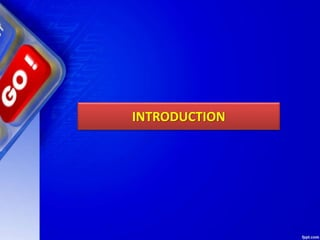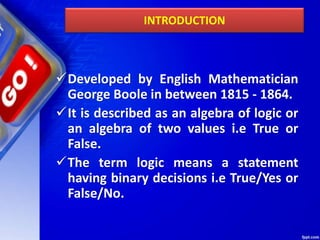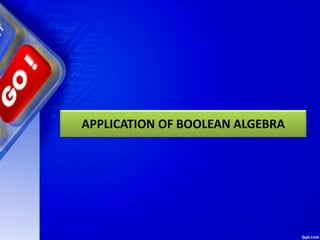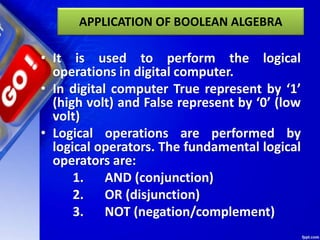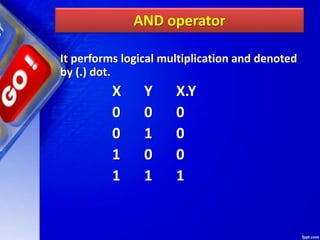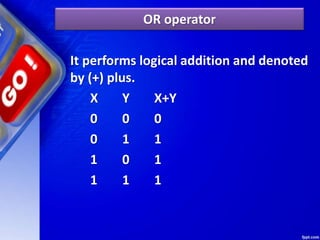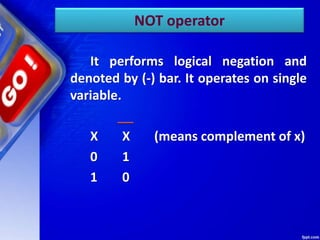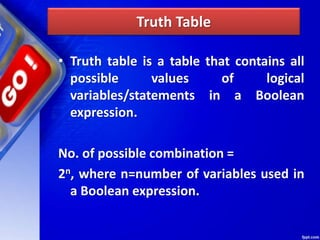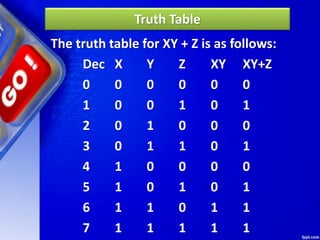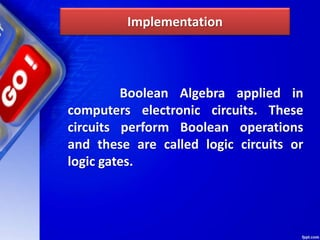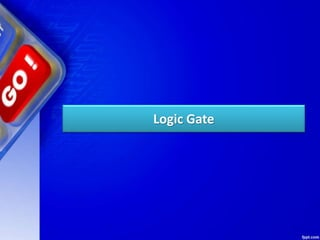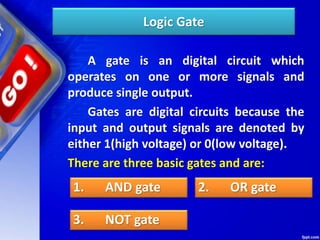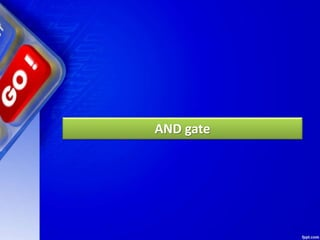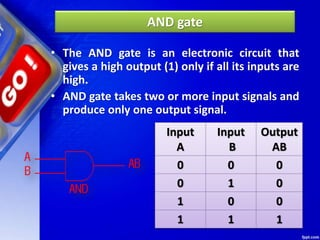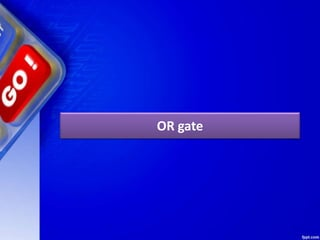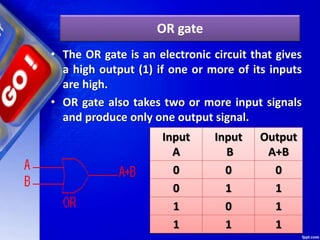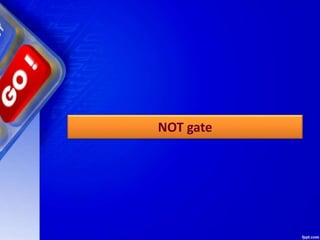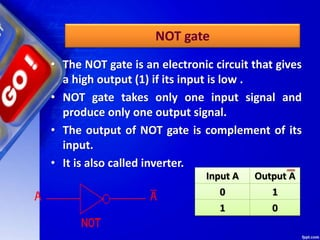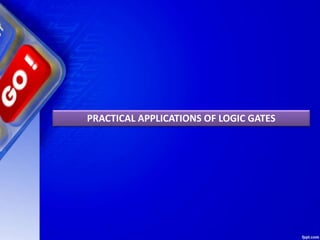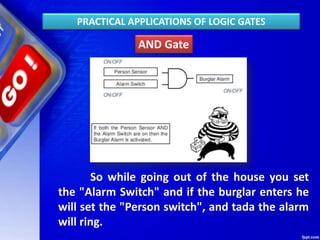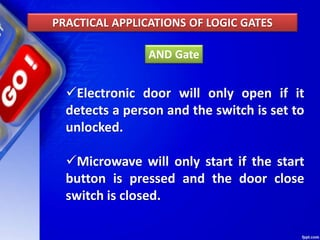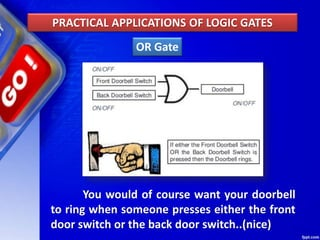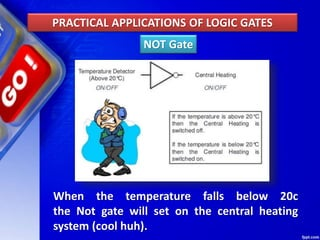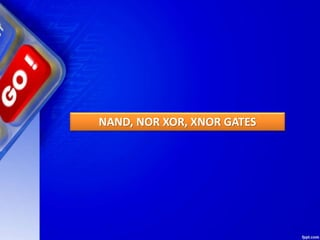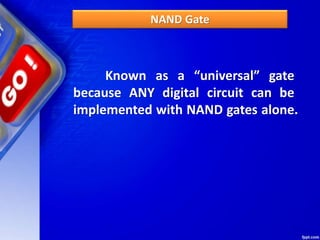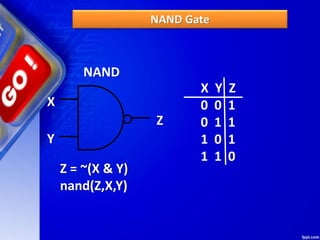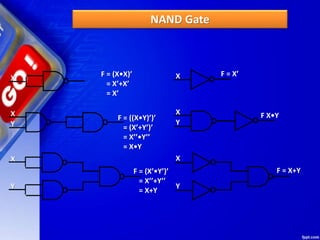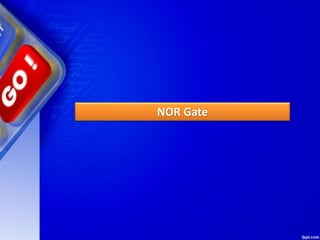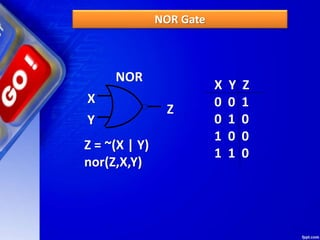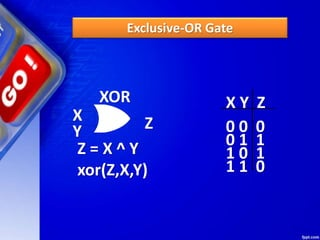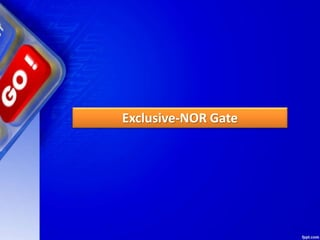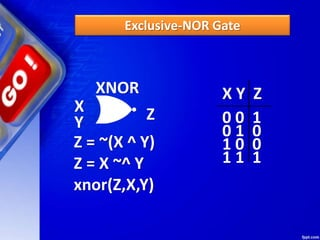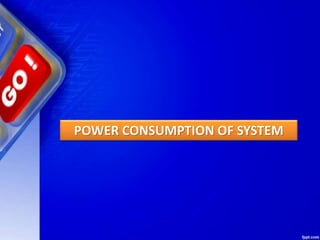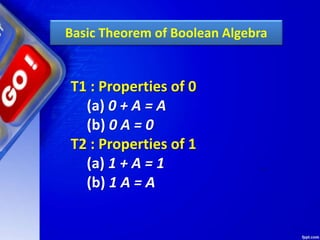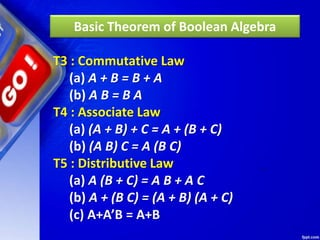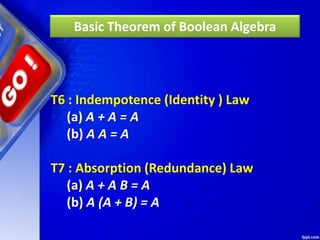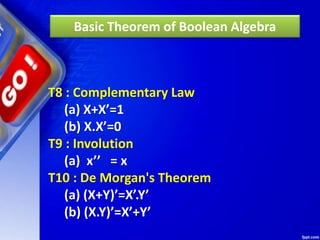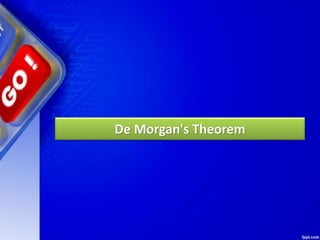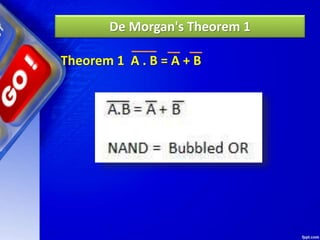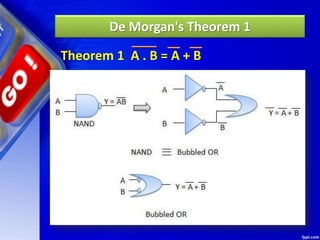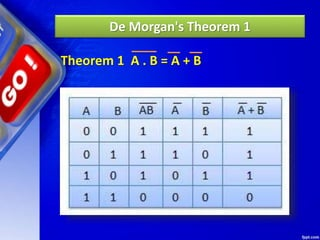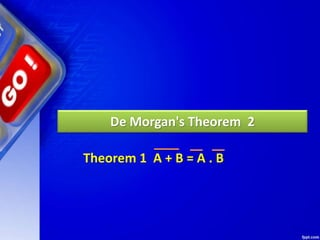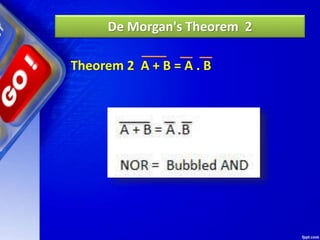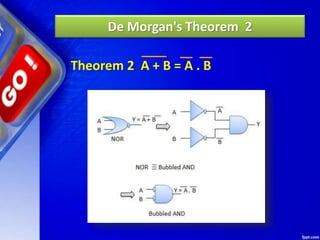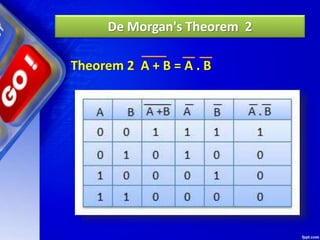1 von 47Chapter 2 Boolean Algebra.pptxHazardRhenz1Chapter 5 boolean algebrapraveenjigajinniBoolean Algebra.pptxASHISHKUMAR15680class xi pptx-1.pptxPriyanshuGumber1Boolean algebrachauhankapilboolean algrebra and logic gates in shortRojin Khadka

### BOOLEAN ALGEBRA & LOGIC GATES.ppt

1. INTRODUCTION
2. INTRODUCTION Developed by English Mathematician George Boole in between 1815 - 1864. It is described as an algebra of logic or an algebra of two values i.e True or False. The term logic means a statement having binary decisions i.e True/Yes or False/No.
3. APPLICATION OF BOOLEAN ALGEBRA
4. APPLICATION OF BOOLEAN ALGEBRA • It is used to perform the logical operations in digital computer. • In digital computer True represent by ‘1’ (high volt) and False represent by ‘0’ (low volt) • Logical operations are performed by logical operators. The fundamental logical operators are: 1. AND (conjunction) 2. OR (disjunction) 3. NOT (negation/complement)
5. AND operator It performs logical multiplication and denoted by (.) dot. X Y X.Y 0 0 0 0 1 0 1 0 0 1 1 1
6. OR operator It performs logical addition and denoted by (+) plus. X Y X+Y 0 0 0 0 1 1 1 0 1 1 1 1
7. NOT operator It performs logical negation and denoted by (-) bar. It operates on single variable. X X (means complement of x) 0 1 1 0
8. Truth Table • Truth table is a table that contains all possible values of logical variables/statements in a Boolean expression. No. of possible combination = 2n, where n=number of variables used in a Boolean expression.
9. Truth Table The truth table for XY + Z is as follows: Dec X Y Z XY XY+Z 0 0 0 0 0 0 1 0 0 1 0 1 2 0 1 0 0 0 3 0 1 1 0 1 4 1 0 0 0 0 5 1 0 1 0 1 6 1 1 0 1 1 7 1 1 1 1 1
10. Implementation Boolean Algebra applied in computers electronic circuits. These circuits perform Boolean operations and these are called logic circuits or logic gates.
11. Logic Gate
12. Logic Gate A gate is an digital circuit which operates on one or more signals and produce single output. Gates are digital circuits because the input and output signals are denoted by either 1(high voltage) or 0(low voltage). There are three basic gates and are: 1. AND gate 2. OR gate 3. NOT gate
13. AND gate
14. AND gate • The AND gate is an electronic circuit that gives a high output (1) only if all its inputs are high. • AND gate takes two or more input signals and produce only one output signal. Input A Input B Output AB 0 0 0 0 1 0 1 0 0 1 1 1
15. OR gate
16. OR gate • The OR gate is an electronic circuit that gives a high output (1) if one or more of its inputs are high. • OR gate also takes two or more input signals and produce only one output signal. Input A Input B Output A+B 0 0 0 0 1 1 1 0 1 1 1 1
17. NOT gate
18. NOT gate • The NOT gate is an electronic circuit that gives a high output (1) if its input is low . • NOT gate takes only one input signal and produce only one output signal. • The output of NOT gate is complement of its input. • It is also called inverter. Input A Output A 0 1 1 0
19. PRACTICAL APPLICATIONS OF LOGIC GATES
20. AND Gate So while going out of the house you set the "Alarm Switch" and if the burglar enters he will set the "Person switch", and tada the alarm will ring. PRACTICAL APPLICATIONS OF LOGIC GATES
21. AND Gate PRACTICAL APPLICATIONS OF LOGIC GATES Electronic door will only open if it detects a person and the switch is set to unlocked. Microwave will only start if the start button is pressed and the door close switch is closed.
22. OR Gate You would of course want your doorbell to ring when someone presses either the front door switch or the back door switch..(nice) PRACTICAL APPLICATIONS OF LOGIC GATES
23. NOT Gate When the temperature falls below 20c the Not gate will set on the central heating system (cool huh). PRACTICAL APPLICATIONS OF LOGIC GATES
24. NAND, NOR XOR, XNOR GATES
25. NAND Gate Known as a “universal” gate because ANY digital circuit can be implemented with NAND gates alone.
26. NAND Gate NAND X Y Z X Y Z 0 0 1 0 1 1 1 0 1 1 1 0 Z = ~(X & Y) nand(Z,X,Y)
27. NAND Gate X X F = (X•X)’ = X’+X’ = X’ X Y Y F = ((X•Y)’)’ = (X’+Y’)’ = X’’•Y’’ = X•Y F = (X’•Y’)’ = X’’+Y’’ = X+Y X X F = X’ X Y Y F X•Y F = X+Y
28. NOR Gate
29. NOR Gate NOR X Y Z X Y Z 0 0 1 0 1 0 1 0 0 1 1 0 Z = ~(X | Y) nor(Z,X,Y)
30. Exclusive-OR Gate
31. Exclusive-OR Gate X Y Z XOR X Y Z 0 0 0 0 1 1 1 0 1 1 1 0 Z = X ^ Y xor(Z,X,Y)
32. Exclusive-NOR Gate
33. Exclusive-NOR Gate X Y Z XNOR X Y Z 0 0 1 0 1 0 1 0 0 1 1 1 Z = ~(X ^ Y) Z = X ~^ Y xnor(Z,X,Y)
34. POWER CONSUMPTION OF SYSTEM
35. Basic Theorem of Boolean Algebra T1 : Properties of 0 (a) 0 + A = A (b) 0 A = 0 T2 : Properties of 1 (a) 1 + A = 1 (b) 1 A = A
36. Basic Theorem of Boolean Algebra T3 : Commutative Law (a) A + B = B + A (b) A B = B A T4 : Associate Law (a) (A + B) + C = A + (B + C) (b) (A B) C = A (B C) T5 : Distributive Law (a) A (B + C) = A B + A C (b) A + (B C) = (A + B) (A + C) (c) A+A’B = A+B
37. T6 : Indempotence (Identity ) Law (a) A + A = A (b) A A = A T7 : Absorption (Redundance) Law (a) A + A B = A (b) A (A + B) = A Basic Theorem of Boolean Algebra
38. T8 : Complementary Law (a) X+X’=1 (b) X.X’=0 T9 : Involution (a) x’’ = x T10 : De Morgan's Theorem (a) (X+Y)’=X’.Y’ (b) (X.Y)’=X’+Y’ Basic Theorem of Boolean Algebra
39. De Morgan's Theorem
40. De Morgan's Theorem 1 Theorem 1 A . B = A + B
41. De Morgan's Theorem 1 Theorem 1 A . B = A + B
42. De Morgan's Theorem 1 Theorem 1 A . B = A + B
43. De Morgan's Theorem 2 Theorem 1 A + B = A . B
44. De Morgan's Theorem 2 Theorem 2 A + B = A . B
45. De Morgan's Theorem 2 Theorem 2 A + B = A . B
46. De Morgan's Theorem 2 Theorem 2 A + B = A . B
47. Thank You